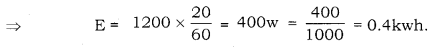# Calculate the electric energy consumed by a 1200W toaster in 20 minutes

Calculate the electric energy consumed by a 1200W toaster in 20 minutes.

We have, E = Pt
It is given that : P = 1200W
We know that, E = P × t
[Where p = electric power and t = time]Hence, Energy consumed = 0.4kwh.